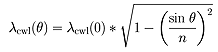Home > Astronomy > INGRID > Narrow Band Filter Transmission Curves

## Narrow Band Filter Transmission Curves

All the filters were scanned at room temperature (~293K) and an angle θ=0° with respect to the incident light. However, the central wavelength of the narrow band filters depends on (i) the angle of incidence and (ii) the temperature, which in the case of INGRID are θ~5° and T~77K respectively. Transmission curves for these values have been estimated from the measured data as follows:
1. The blueshift resulting from the decrease in temperature (from 293K to 77K) was estimated using the change of CWL (central wavelength) per Kelvin given by the manufacturer (see column 9 of the narrow band filter table).
1. The effect of the 5° tilt on the central wavelength was then taken into account using the following equation, which relates the CWL at angle θ to that at 0° incidence, for an interference filter of effective refraction index n. A value of n=2 was assumed for all the filters (see Ghinassi et al. 2002, A&A, 386, 1157).An increase in transmission of about ~6-8% is expected for the filters at T=77K, which has not been taken into account in the calculation.

 Top | BackContact:  (INGRID Instrument Specialist)Last modified: 17 June 2012